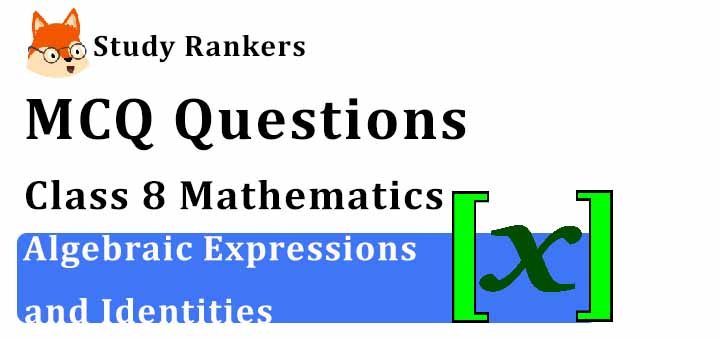## MCQ Questions for Class 8 Maths: Ch 9 Algebraic Expressions and Identities1. Expressions consists of _____________ and _______________.
(a) variables, constants
(b) identities
(c) expressions
(d) none of these
► (a) variables, constants

2. Which of the following is an expression?
(a) 1/2
(b) 3
(c) 3x-2
(d) 2
► (c) 3x-2

3. The coefficient of x in the expression -7x +5 is
(a) 5
(b) -7
(c) 7
(d) 0
► (b) -7

4. The number of terms in the expression 2x2+3x+5 is
(a) 1
(b) 2
(c) 3
(d) 5
► (c) 3

5. Like terms in the expression 7x,5x2,7y, -5yx, -9x2, are
(a) 7x, -5yx
(b) 5x2, -5yx
(c) 5x2, -9x2
(d) 7x, 7y
► (c) 5x2, -9x2

6. Terms are added to form ___________.
(a) expressions
(b) terms
(c) identities
(d) none of these
► (a) expressions

7. Add: 7xy + 5yz – 3zx, 4yz + 9zx – 4y, –3xz + 5x – 2xy.
(a) 5xy + 3zx + 5x – 4y
(b) 5xy + 9yz +2zx + 5x – 4y
(c) 5xy + 9yz +3zx + 5x – 4y
(d) 5xy + 9yz +3zx + 4y
► (c) 5xy + 9yz +3zx + 5x – 4y

8. The expression x + y + z is in
(a) one variable
(b) no variable
(c) three variables
(d) two variables
► (c) three variables

9. Which of the following is a monomial ?
(a) 4x²
(b) a + 6
(c) a + 6 + c
(d) a + b + c + d
► (a) 4x²

10. How many terms are there in the expression 5 – 3xy ?
(a) 1
(b) 2
(c) 3
(d) 5
► (b) 2

11. How many terms are there in the expression 5xy + 9yz + 3zx + 5x – 4y ?
(a) 1
(b) 3
(c) 4
(d) 5
► (d) 5

12. If x = 3 is solution of x² + kx + 15, value of k is
(a) k = -8, x = 3
(b) k = 8, x = 5
(c) k = 6, x = 5
(d) None of above
► (a) k = -8, x = 3

13. The coefficient in the term -5x is
(a) 5
(b) -5
(c) 1
(d) 2
► (b) -5

14. n (4 + m) = 4n + ___
(a) 4m
(b) 4n
(c) 4mn
(d) nm
► (d) nm

15. The like terms of the following are
(a) x, 3x
(b) x, 2y
(c) 2y, 6xy
(d) 3x, 2y
► (a) x, 3x

16. Value of expression ‘a(a2+a +1)+5’ for ‘ a’ = 0 is
(a) a+5
(b) 1
(c) 6
(d) 5
► (d) 5

17. Which of the following is like term as 7xy?
(a) 9
(b) 9x
(c) 9y
(d) 9xy
► (d) 9xy

18. The area of triangle is’ xy’ where’ x’ is length and ‘y’ is breadth. If the length of rectangle is increased by 5 units and breadth is decreased by 3 units, the new area of rectangle will be
(a) (x-y)(x+3)
(b) (xy+15)
(c) (x+5)(y-3)
(d) (xy+5-3)
► (c) (x+5)(y-3)

19. The expression 7xy has the factors
(a) 7,x,y
(b) x,y
(c) 7,x
(d) 7,y
► (a) 7,x,y

20. Which of the following is a binomial?
(a) 4x + y + 2
(b) 2x + 7
(c) 3x + 4y - 6
(d) 3x
► (b) 2x + 7

21. When numbers/literals are added or subtracted, they are called _________.
(a) identities
(b) expressions
(c) variables
(d) terms
► (d) terms

22. The volume of rectangular box whose length, breadth and height is 2p,4q.8r respectively is
(a) 14pqr
(b) 2p+4q+8r
(c) 64pqr
(d) 64
► (c) 64pqr